# Indices and roots

### Term 3 starting in week 11 :: Estimated time: 2 weeks

• Square and Cube numbers (review)
• Calculate higher powers and roots
• Powers of ten and standard form (review)
• The addition and subtraction rules for indices (review)
• Understand and use the power zero and negative indices
• Work with powers of powers
• Calculate with numbers in standard form (review)

For higher-attaining pupils:

• Understand and use fractional indices

This page should remember your ticks from one visit to the next for a period of time. It does this by using Local Storage so the information is saved only on the computer you are working on right now.

## Lesson Starters

Here are some suggestions for whole-class, projectable resources which can be used at the beginnings of each lesson in this block.

### 1st Lesson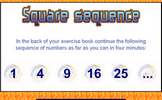#### Square Sequence

Write out as many square numbers as possible in 4 minutes.

### 2nd Lesson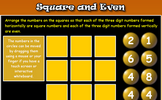#### Square and Even

Arrange the numbers on the cards so that each of the three digit numbers formed horizontally are square numbers and each of the three digit numbers formed vertically are even.

### 3rd Lesson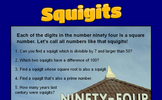#### Squigits

A challenge to find numbers which have each of their digits as square numbers.

### 4th Lesson#### Exponential Error

This lesson starter presents a number of statements about indices and pupils are asked if they can spot the mistake.

### 5th Lesson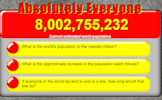#### Absolutely Everyone

A choice of questions about the current world population which is constantly changing.

### 6th Lesson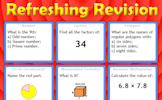#### Refreshing Revision

It is called Refreshing Revision because every time you refresh the page you get different revision questions.

Some of the Starters above are to reinforce concepts learnt, others are to introduce new ideas while others are on unrelated topics designed for retrieval practice or and opportunity to develop problem-solving skills.

White Rose ResourcesEnd of block assessments provide a quick progress check at the end of each block of learning to make sure students have understood the content covered. This Scheme of Learning was produced by White Rose Maths and is used here with permission granted on 30th June 2021.For All: